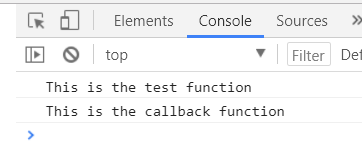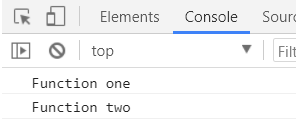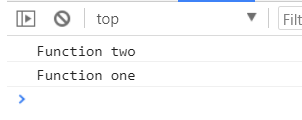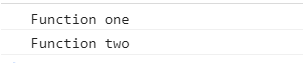# JavaScript Callback Functions

Leave a comment

A callback function in JavaScript is a function passed to another function that is then called after an event has occurred. This is useful when you don’t know how much time the function will take to complete, such as a call to a web API.

In the simple example below, we have a function called callback() that writes to the console. We then have a function called testCallback() that calls the callback function:

```function callback() {
console.log("This is the callback function");
}

function testCallback(func) {
console.log("This is the test function");
func();
}

testCallback(callback);
```

This produces:Now, let’s look at how JavaScript executes functions. If we have 2 functions, one and two:

```function one() {
console.log("Function one");
}

function two(func) {
console.log("Function two");
}

one();
two();
```

This produces:Now what if function one is a call to an API that takes some time to execute. What would happen, if we would could see the reverse output, where function two finishes executing before function one. This can cause problems if we are wanting our code to execute sequentially and based on a result of function one executing.

For example:

```function one() {
// Do something that takes time
setTimeout(function() {
console.log("Function one")}, 5000);
}

function two() {
console.log("Function two");
}

one();
two();
```

This would produce “Function two”, then wait for 5 seconds, then print “Function one”:To fix this, we can use a callback. Below we are passing function two as a callback to function one. Once Function one, which is the “higher order” function, has finished its asynchronous call, we will be able to run function two:

```function one(callback) {
// Do something that takes time
setTimeout(function() {
console.log("Function one");
callback();
}, 5000);
}

function two() {
console.log("Function two");
}

one(function() {two()});
```

So the output is produced after a 5 second wait, then both statements printed:As mentioned, this is a useful way of ensuring code can continue to execute, so the UI isn’t tied up waiting for a process to complete.

THANKS FOR READING. BEFORE YOU LEAVE, I NEED YOUR HELP.

I AM SPENDING MORE TIME THESE DAYS CREATING YOUTUBE VIDEOS TO HELP PEOPLE LEARN THE MICROSOFT POWER PLATFORM.

IF YOU WOULD LIKE TO SEE HOW I BUILD APPS, OR FIND SOMETHING USEFUL READING MY BLOG, I WOULD REALLY APPRECIATE YOU SUBSCRIBING TO MY YOUTUBE CHANNEL.

THANK YOU, AND LET'S KEEP LEARNING TOGETHER.

CARL

ABOUT CARL DE SOUZACarl de Souza is a developer and architect focusing on Microsoft Dynamics 365, Power BI, Azure, and AI.

carldesouza.comLinkedIn Twitter | YouTube